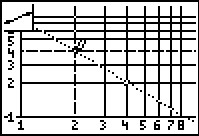# Activities

••• ##### Subject Area

• Math: Algebra II: Logarithmic Functions

• ##### Author9-12

45 Minutes

• ##### Device
• TI-83 Plus Family
• TI-84 Plus
• TI-84 Plus Silver Edition
•TI-84 Plus C Silver Edition
•TI-84 Plus CE
• ##### Software

TI Connect™
TI Connect™ CE

• ##### Accessories

TI Connectivity Cable

• ##### Report an Issue

Properties of Logarithms#### Activity Overview

Students use a combination of algebra and graphing to discover the properties of logarithms.

#### Key Steps

•In Problem 1, students investigate the power property of logarithms. Students use Lists to calculate the value of b = a2 for positive integral values of a.

Next, students define two new variables x = log a and y = log b and enter them into lists. Graphing this data in a scatter plot, students can use the LinReg command to determine that the graph of log a2 resembles the linear equation y = 2x. Rewriting this as a logarithmic equation, students obtain log a2 = 2 log a, revealing the Power Property of Logarithms.

•In Problem 2, students investigate the product property of logarithms. Students will again use Lists to do their investigation, this time to find the value of b = 6a, for given values of a.

Students graph the data in a scatter plot and find the y-intercept. Students should realize that this value is log 6. They can then rewrite the equation y = log 6 + x in terms of a. This will reveal the Product Property of Logarithms.

•Problem 3 gives students the opportunity to investigate the Quotient Property of logarithms. Students experiment with a logarithmic scale on both the x- and y-axes.